# Workarounds To Sum Data While Using NONE as Summary Method Within Source Moduleedited November 2022

As the Anaplan model size capacity has recently grown from roughly 18 billion cells to 100 billion cells with the introduction of HyperModel, it is still important to structure models efficiently, while creating as little 'wasted space' as possible.

The Planual states that the Summary Method for most line items should be set to None. This is a great rule to follow—unless:

• The totals need to be displayed on a dashboard/page.
• The data needs to be aggregated.

Let's assume we have several levels in a cost center hierarchy as shown below:

- L1 Region

- L2 Sub Region

- L4 Cost Center

Let's assume we have a source module dimensioned by Accts, L4 Cost Centers, Months, and a single line item where NONE is the Summary Method.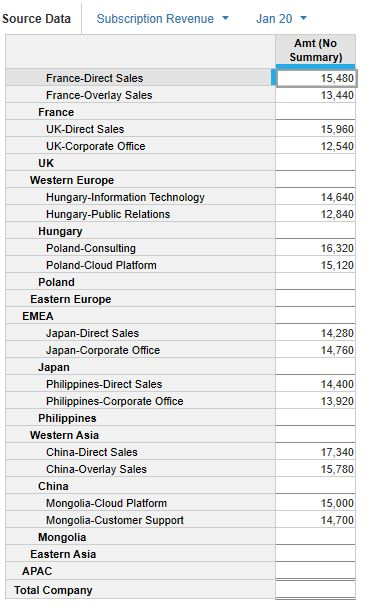Now, let's say we have a target module dimensioned by Accts, L2 Sub Region, Months, and some line items.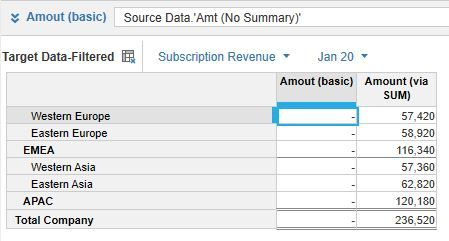If we simply reference the source module without any functions then this will not roll the data up (see above line item on the left). A quick way around this is to use the SUM formula and reference an SYS module that has the mapping of the L4 Cost Centers to the L3, L2, and L1.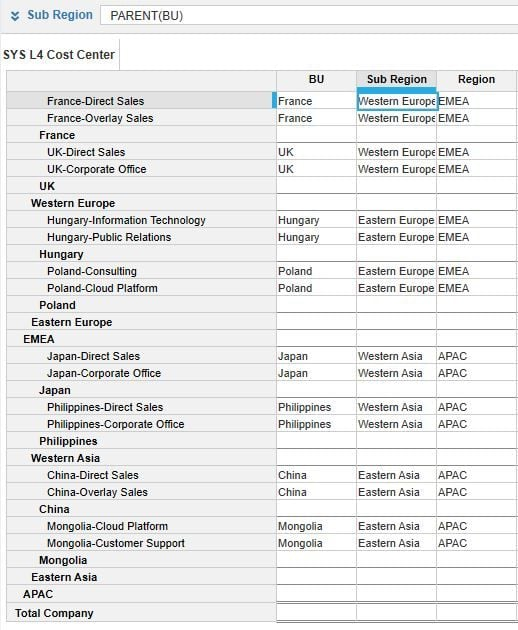In this example, the formula would be Source Data.'Amt (No Summary)'[SUM: 'SYS L4 Cost Center'.Sub Region]. Simple enough, right?

But what if the target module does not contain a higher level dimension of the L4 Cost Center structure? A workaround is to create a one-item dimension...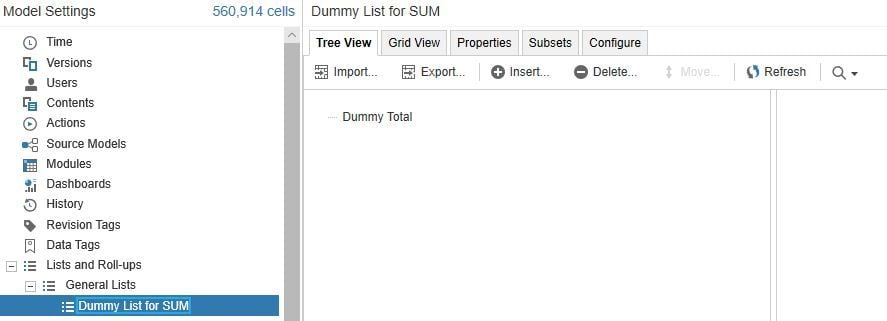...and map it to the L4 Cost Centers list within the L4 Cost Center SYS module.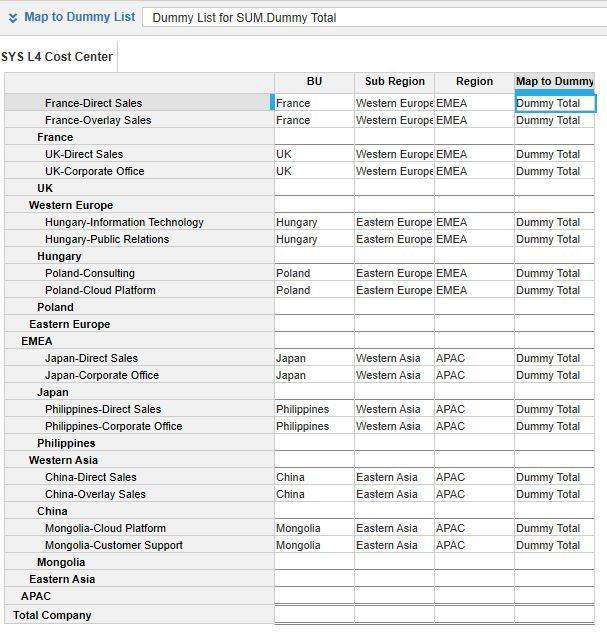Whereas the L4 Cost Center mapping to L3, L2, etc. is based on the composite structure and uses the PARENT formula, the mapping to this one-item list is just OneItemList.MemberOfOneItemList.

Then, in order to sum the data within a target module, the one-item dimension must be included in the structure and reference the L4 Cost Center SYS module for the one-item mapping via a SUM formula.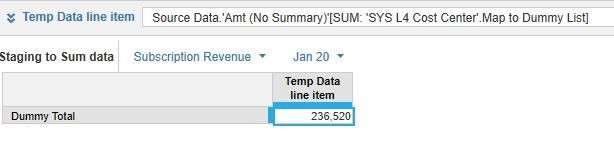If it is not desired to have the one-item dimension in the final output module, then the above module can be used as the source in conjunction with a line item to select the 'Dummy Total'...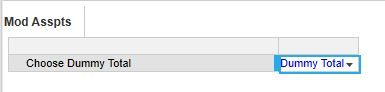...and then feed into a target model that references the staging module using a LOOKUP statement to point to the above line item.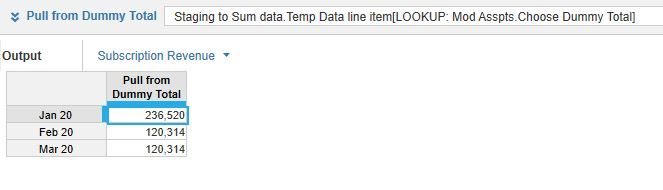Depending on the number of levels and size of the dimensions for the source and target modules this can save a lot of space, roughly 30-70%. For small modules, using this approach is likely not worth the effort, but this can provide tremendous space savings on very large modules.

Tagged:

•edited November 2022

How would you sum months into years without summary method?

•edited November 2022

Hello,

Thank you for sharing this!

What is the difference of impact on performance when we use this aggregation method with SUM vs. when we use native summary method?

Thank you!

Amy

•edited November 2022

Hi @AmyX

Here is some analysis I did for a client (it depends on the how many updates are being made and how many of the cells are 0). Dummy sum vs native summation - performance considerations:

• When loading data/opening a model, because there are less subtotals to calculate with the Dummy Sum you are reducing the number of calculations that are run. This can improve performance.
• However, when processing a user update (which is often only a few cells in the source data), the Native Summation can leverage the subtotals and only calculate updated cells. Meaning less calculations are run and improved performance.
• Native Summation has certain optimisations built into it, for example when the cells being totalled are empty/0 subsequent calculations aren't run. In this case the Native Summation will run a lot faster than the Dummy Sum which doens't benefit from the same optimisation.
•edited November 2022

To sum months into years you can use MOVINGSUM(Line item, 0,0)

Still, I'm not sure whether the tradeoff between saving model space and the readability of the model pays off in this situation.

This approach could save almost 50% of the required space (12 months vs. 12m + 4q + 1y), which is worth trying for large modules. Nevertheless, another concern for the large modules is calculation time, which should be tested case by case. I assume the native YEARVALUE() would show better results than the artificially built formula.

•edited November 2022

Thank you, @whitby